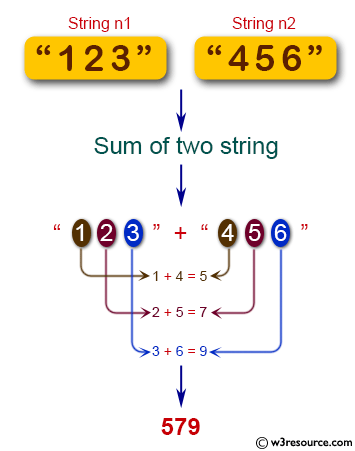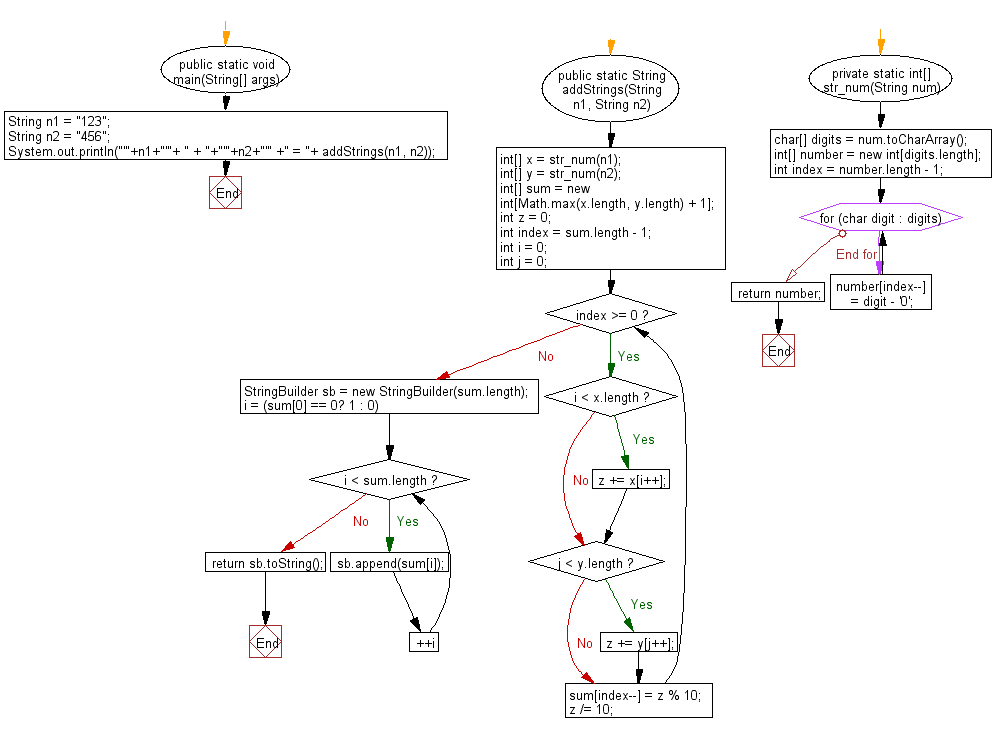﻿ Java programming exercises: Given two non-negative integers represented as string, return their sum - w3resource# Java Exercises: Given two non-negative integers represented as string, return their sum

## Java Basic: Exercise-189 with Solution

Write a Java program to given two non-negative integers num1 and num2 represented as string, return the sum of num1 and num2.

Pictorial Presentation:Sample Solution:

Java Code:

``````import java.util.*;
public class Solution {
public static void main(String[] args) {
String n1 = "123";
String n2 = "456";
System.out.println("'" + n1 + "'" + " + " + "'" + n2 + "'" + " = " + addStrings(n1, n2));
}
public static String addStrings(String n1, String n2) {
int[] x = str_num(n1);
int[] y = str_num(n2);
int[] sum = new int[Math.max(x.length, y.length) + 1];
int z = 0;
int index = sum.length - 1;
int i = 0;
int j = 0;
while (index >= 0) {
if (i < x.length) {
z += x[i++];
}
if (j < y.length) {
z += y[j++];
}
sum[index--] = z % 10;
z /= 10;
}
StringBuilder sb = new StringBuilder(sum.length);
for (i = (sum == 0 ? 1 : 0); i < sum.length; ++i) {
sb.append(sum[i]);
}
return sb.toString();
}

private static int[] str_num(String num) {
char[] digits = num.toCharArray();
int[] number = new int[digits.length];
int index = number.length - 1;
for (char digit: digits) {
number[index--] = digit - '0';
}
return number;
}
}
```
```

Sample Output:

```'123' + '456' = 579
```

Flowchart:Java Code Editor:

Company:

What is the difficulty level of this exercise?

﻿

## Java: Tips of the Day

Array vs ArrayLists:

The main difference between these two is that an Array is of fixed size so once you have created an Array you cannot change it but the ArrayList is not of fixed size. You can create instances of ArrayLists without specifying its size. So if you create such instances of an ArrayList without specifying its size Java will create an instance of an ArrayList of default size.

Once an ArrayList is full it re-sizes itself. In fact, an ArrayList is internally supported by an array. So when an ArrayList is resized it will slow down its performance a bit as the contents of the old Array must be copied to a new Array.

At the same time, it's compulsory to specify the size of an Array directly or indirectly while creating it. And also Arrays can store both primitives and objects while ArrayLists only can store objects.

Ref: https://bit.ly/3o8L2KH